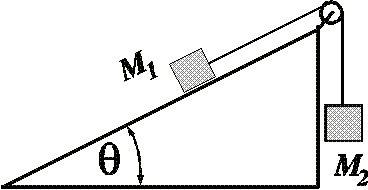# Frictionless Incline Problem

• NoobeAtPhysics

## Homework Statement

M1 and M2 are two masses connected as shown. The pulley and the incline are frictionless. Find the mass M1, given that M2 (4.00 kg) accelerates downwards at 3.53 m/s2, and that θ = 32.0°.F = m*a
Fk = u*N

## The Attempt at a Solution

Forces on m1

Fy = T - N - m1*g*sin(theta) = m1*a
Fx = N - m1*g*cos(theta) = 0

Forces on m2

Fy = m2*g*T = m2*a

Using equations above,

T = m2(g-a)
N = m1*g*cos(theta)

m2*(g-a) - m1*g*cos(theta)-m1*g*sin(theta) = m1*a

rearranging for m1

m1 =[m2*(g-a)]/[a+g(sin(theta) + cos(theta))]

Plugging in the correct values, I get 1.47kg for my answer which is... wrong :(

Thank you,
Noobe

I get

T - sin(θ)m1 g = m1 a
T - m2 g = -m2 a

Two equation with 2 unknowns. Does that help ?

Last edited:
•1 person
Thank you Mentz that is very helpful! I get the right answer when I use your equations. Thank you again. =]

Question:

T - m2 g = -m2 a

Why is it -m2 a and not just m2 a?

Thank you Mentz that is very helpful! I get the right answer when I use your equations. Thank you again. =]

Question:

T - m2 g = -m2 a

Why is it -m2 a and not just m2 a?

Think about Newton's second law: F=ma
What is the acceleration of m1? What is the acceleration of m2?
consider gravity and the coordinate system Mentz114 has chosen when drawing free body diagrams for both blocks. That should answer your question.

•1 person
Thank you very much guys, I understand it now! :), Physics is fun